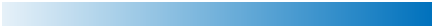# sky

Sky colormap array

Since R2023a

•## Syntax

``c = sky``
``c = sky(m)``

## Description

example

````c = sky` returns the sky colormap as a three-column array with the same number of rows as the colormap for the current figure. If no figure exists, the number of rows is 256. Each row in the array contains the red, green, and blue intensities for a specific color. The intensities are in the range [0, 1], and the color scheme looks like this image.```

example

````c = sky(m)` returns the colormap with `m` colors.```

## Examples

collapse all

Plot a surface and assign the sky colormap.

```surf(peaks) colormap("sky")```Get the sky colormap array and reverse the order. Then apply the modified colormap to the surface.

```c = sky; c = flipud(c); colormap(c)```Get a downsampled version of the sky colormap containing only twenty colors. Then display the contours of the peaks function by applying the colormap and interpolated shading.

```c = sky(20); surf(peaks) colormap(c) shading interp```Heatmap charts use the 256-sampling of the `sky` colormap by default. You can change the sampling by specifying the `Colormap` name-value argument.

For example, create two heatmap charts in a 2-by-1 tiled chart layout. The top chart uses the default set of 256 colors, and the bottom chart uses only two colors.

```tiledlayout(2,1,TileSpacing="compact") % Top chart with default colors nexttile heatmap(magic(10)) % Bottom chart with two colors nexttile heatmap(magic(10),Colormap=sky(2))```## Input Arguments

collapse all

Number of colors, specified as a positive number. The default value of `m` is the number of colors in the current figure's colormap. If no figure exists, the default value is 256.

Data Types: `single` | `double`

## Version History

Introduced in R2023a